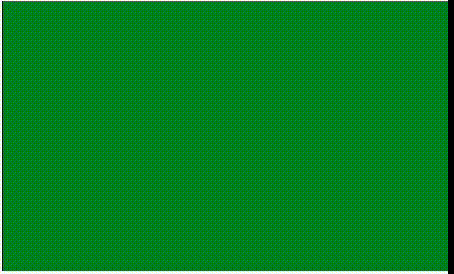# p5.js | redraw() Function

• Last Updated : 23 Aug, 2019

The redraw() function is used to execute the code within draw() function one time. This functions is used to update the display window only when it necessary. The redraw() function does not work when it is called inside the draw() function. The loop() and noLoop() function is used to enable/disable the animations.

Syntax:

`redraw( n )`

Parameters: This function accepts single parameter n which is used to set the redraw for n times. The default value of this function is 1.

Below example illustrates the redraw() function in p5.js:

Example:

 `let l = 0;`` ` `function` `setup() {``   ` `  ``// Create canvas of given size``  ``createCanvas(500, 300);``   ` `  ``// Set the background color``  ``background(``'green'``);`` ` `}`` ` `function` `draw() {``   ` `  ``// Set the stroke color``  ``stroke(``'black'``);``   ` `  ``// Function to draw the line``  ``line(l, 0, l, height);``   ` `}`` ` `function` `mousePressed() {``  ``l += 1;``  ``redraw();``}`

Output:My Personal Notes arrow_drop_up